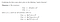# Master Theoremhttps://math.stackexchange.com/q/646908

# EXAMPLE #1

T(n) = T(2n/3) + 1
T(0) = 0

T(n) = T(2n/3) + 1
= 1*T(n / (3/2) ) + n⁰

so a=1, b= (3/2) , d=0

# EXAMPLE #2

T(n) = 2T(n/2) + n log n

T(n) = 2T(n/2) + n log n

T(n) = AT(n/B) + f(n)

So A= 2, B=2, f(n) = n¹ log¹ n → D=1 and K=1

# EXAMPLE #3

T(n) = 2T(n/2) + Θ( n )

T(n) = 2T(n/2) + Θ( n )

T(n) = 2T(n/2) + n

T(n) = 2T(n/2) + n¹

T(n) = AT(n/B) + f(n)

A=2, B=2, f(n) = n¹ → D=1, K=0

# Udemy Videos on Algortithm Analysis:

Written by

## randerson112358

#### A programmer who loves computer science, math, machine learning, data analytics and basketball ! YouTube Channel: https://www.youtube.com/user/randerson112358

Welcome to a place where words matter. On Medium, smart voices and original ideas take center stage - with no ads in sight. Watch
Follow all the topics you care about, and we’ll deliver the best stories for you to your homepage and inbox. Explore
Get unlimited access to the best stories on Medium — and support writers while you’re at it. Just \$5/month. Upgrade